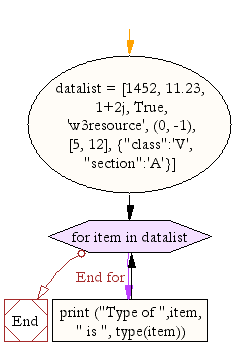﻿ Python Exercise: Prints each item and its corresponding type from a list - w3resource# Python Exercise: Prints each item and its corresponding type from a list

## Python Conditional: Exercise-7 with Solution

Write a Python program that prints each item and its corresponding type from the following list.

Sample List : datalist = [1452, 11.23, 1+2j, True, 'w3resource', (0, -1), [5, 12], {"class":'V', "section":'A'}]

Sample Solution:

Python Code:

``````datalist = [1452, 11.23, 1+2j, True, 'w3resource', (0, -1), [5, 12],
{"class":'V', "section":'A'}]
for item in datalist:
print ("Type of ",item, " is ", type(item))
```
```

Sample Output:

```Type of  1452  is  <class 'int'>
Type of  11.23  is  <class 'float'>
Type of  (1+2j)  is  <class 'complex'>
Type of  True  is  <class 'bool'>
Type of  w3resource  is  <class 'str'>
Type of  (0, -1)  is  <class 'tuple'>
Type of  [5, 12]  is  <class 'list'>
Type of  {'class': 'V', 'section': 'A'}  is  < class 'dict'>
```

Flowchart:## Visualize Python code execution:

The following tool visualize what the computer is doing step-by-step as it executes the said program:

Python Code Editor:

Have another way to solve this solution? Contribute your code (and comments) through Disqus.

What is the difficulty level of this exercise?

Test your Python skills with w3resource's quiz

﻿

## Python: Tips of the Day

Returns a list with n elements removed from the beginning

Example:

```def tips_take(itr, n = 1):
return itr[:n]
print(tips_take([1, 2, 3], 5))
print(tips_take([1, 2, 3], 0))
```

Output:

```[1, 2, 3]
[]
```